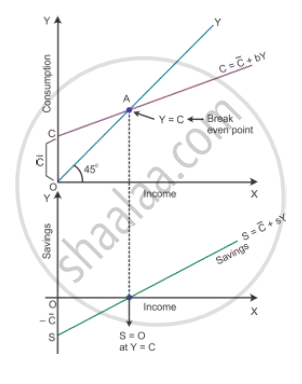# Given Consumption Curve, Derive Saving Curve and State the Steps Taken in the Process of Derivation. Use Diagram. - Economics

Given consumption curve, derive saving curve and state the steps taken in the process of derivation. Use Diagram.

Outline the steps required to be taken in deriving saving curve from the given consumption curve. Use diagram

Give a consumption curve, outline the steps required to be taken in deriving a saving curve from it. Use diagram

Outline the steps in the deriving saving curve from the given consumption curve. Use Diagram.

#### Solution

In the diagram, the consumption curve is given as barC+bY, where barC represents the autonomous consumption, Y is income and b is the rate at which C increases corresponding to an increase in Y. The aggregate supply curve is the 45° line. Consumption is equal to income at Point E.Derivation of saving function from consumption function:

-barC is the saving function where negative savings are equal to autonomous consumption at Y = 0. This is shown on the negative axis in the lower panel at Point S. Here, all the income is spent on consumption expenditure. Hence, there is no saving which is shown as the breakeven point. After this point, S and Y are joined to have a straight line sloping curve.

Concept: Concept of Aggregate Demand and Aggregate Supply
Is there an error in this question or solution?